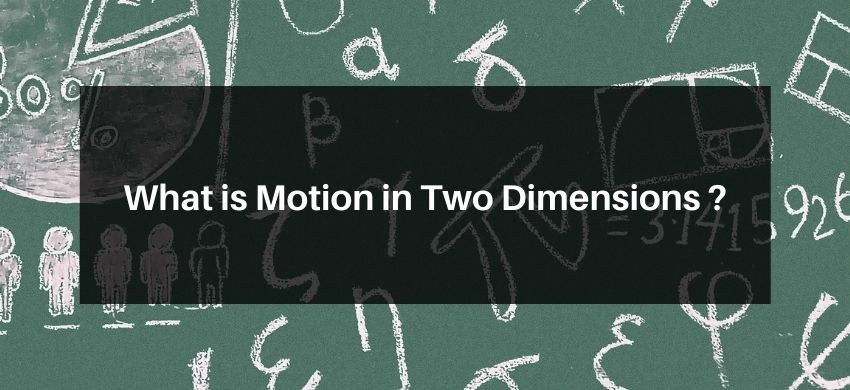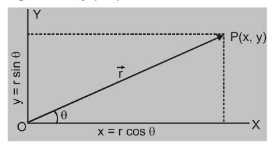# What is Motion in Two Dimensions ?## Motion in Two Dimensions

An object moving in a plane is said to have two-dimensional motion. The two-dimensional motion is equal to the vector sum of two one-dimensional motions along the mutually perpendicular direction.

Let the position of point $P$ at a time $t$ be given by position

vector $\overrightarrow{\mathrm{OP}}=\overrightarrow{\mathrm{r}}$

$\vec{r}=\hat{i} r \cos \theta+\hat{j} r \sin \theta=\hat{i} x+\hat{j} y$